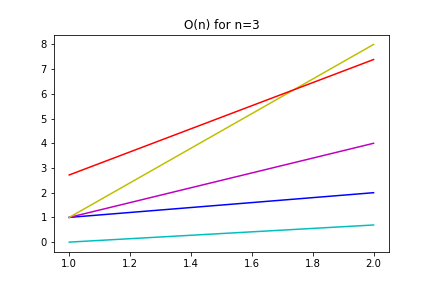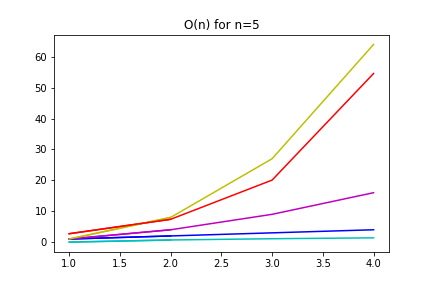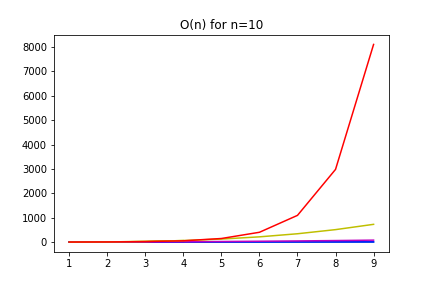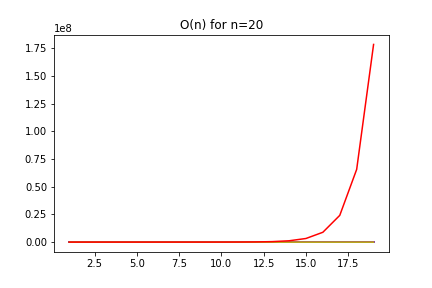# Python | Visualizing O(n) using Python

• Difficulty Level : Hard
• Last Updated : 17 Sep, 2019

## Introduction

Algorithm complexity can be a difficult concept to grasp, even presented with compelling mathematical arguments. This article presents a tiny Python program that shows the relative complexity of several typical functions. It can be easily adapted to other functions.

## Complexity. Why it matters?

Computational complexity is a venerable subject in Computer Science. It can be defined as the amount of time and space that an algorithm needs to solve an instance of a problem.

Attention geek! Strengthen your foundations with the Python Programming Foundation Course and learn the basics.

To begin with, your interview preparations Enhance your Data Structures concepts with the Python DS Course. And to begin with your Machine Learning Journey, join the Machine Learning - Basic Level Course

The underpinnings of computational complexity are mathematical, but its implications very practical. There are problems that are “intractable”. They are not impossible (i.e. undecidable) but no efficient algorithm is known for them. That is: they are very difficult to solve with today technology, and even with the foreseeable one.

## Usually, worst-case is the best

The most popular analysis in computational complexity is the worst-case scenario. Despite its pessimism, it is very reasonable: the size of the problems willing to be solved increases as time goes. We want to process petabytes of data, instead of megabytes. So, size is an all-important factor in algorithm complexity.

Consider the input size as the independent variable, and the growth rate is the dependent variable, and try to analyze its performance as the input size grows to infinity. This analysis is called big-Oh and has many rules that you can consult in any good algorithmic textbook. The most important one is that constants do not affect algorithmic performance for large inputs. The reason, again, is that the size of the input is the most important factor and constants do not depend on the input size.

## Comparing function growths in Python

Newcomers to the theory of computation often get confused by the fact that exponential functions likeare worse than polynomial functions like, say. This is clear from the mathematical definition of the Big-Oh function, but it is not easy to see unless we think that we account for very large.

The following Python code visualizes the growth as the problem instance (N) increases of several functions:. Note thatis considered a bad performance as it takesoperations to process 1000 inputs. In general,is considered bad for k>=2.

The code uses the libraries NumPy and MatPlotLib and employs a functional programming technique called currying to computefor constant k. It is easy to compute other functions by modifying the list FUNCTIONS.

Code : Python code explaining asymptotic behaviour of several functions.

 `# Python code that compares the ``# asymptotic behaviour of several functions`` ` `import` `numpy as np``import` `matplotlib.pyplot as plt`` ` `# Returns a function that computes x ^ n for a given n``def` `poly(n):``    ``def` `polyXN(x):``        ``return` `x``*``*``n``    ``return` `polyXN`` ` `# Functions to compare and colors to use in the graph``FUNCTIONS ``=` `[np.log, poly(``1``), poly(``2``), poly(``3``), np.exp]``COLORS ``=` `[``'c'``, ``'b'``, ``'m'``, ``'y'``, ``'r'``]`` ` `# Plot the graphs ``def` `compareAsymptotic(n):``    ``x ``=` `np.arange(``1``, n, ``1``)``    ``plt.title(``'O(n) for n ='``+``str``(n))``    ``for` `f, c ``in` `zip``(FUNCTIONS, COLORS):``        ``plt.plot(x, f(x), c)``    ``plt.show()``         ` `compareAsymptotic(``3``)``compareAsymptotic(``5``)``compareAsymptotic(``10``)``compareAsymptotic(``20``)`

The results are not surprising: the exponential function has the worst performance, as it grows very quick given the input size. For N=20, the other functions are insignificant compared with the exponential.

The logarithm is shown in cyan, the polynomials in blue, magenta and yellow and the exponential in red.My Personal Notes arrow_drop_up